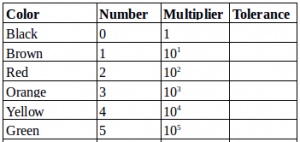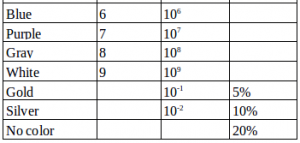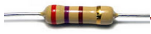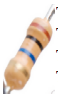# Resistor color code

### Article about the Resistor color code

The resistor is one component of an electrical circuit that functions to control the number of electric currents. In general, there are two types of resistors, namely wire coil resistors and carbon resistors. Wire roll resistors are usually used in the laboratory, made by wrapping fine wire on the surface of the insulator tube. Carbon resistors are typically used in electronic circuits, cylindrical, and have wires at both ends. The value of the carbon resistor resistance is expressed in color code and displayed on the surface of the resistor.

The resistance value of a resistor can be known by interpreting the resistor color code. To understand this, first look at the following table, then study the example problem to determine the resistor resistance value.Sample problem 1:

The resistor resistance value on the figure is …

Solution:The first two colors represent the first two numbers of the resistance value, the third color represents the tenth power used to multiply, and the fourth color represents the tolerance of deviation.

The first color = red = 2

The second color = orange = 3

The third color = brown = 101 = 10

The fourth color = gold = 5%

The resistor value is 230 Ω with a deviation of 5% x 230 Ω = 11.5 Ω. If there is a change in temperature, the minimum resistance is 230 11 – 11.5 Ω = 218.5 Ω and the maximum resistance is 230 Ω + 11.5 Ω = 241.5 Ω.

Sample problem 2:

What is the value of the resistor resistance in the figure?

Solution:The first color = brown = 1

The second color = black = 0

The third color = blue = 106 = 1000,000

The fourth color = gold = 5%

The resistor value is 10,000,000 .000 with a deviation of 5% x 10,000,000 Ω = 500,000 Ω. If there is a change in temperature, the minimum obstacle is 10,000,000 500 – 500,000 Ω = 9,500,000 Ω and the maximum obstacle is 10,000,000 Ω + 500,000 Ω = 10,500,000 Ω.